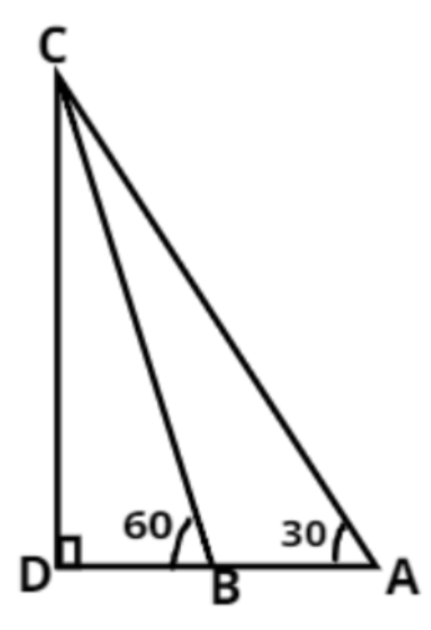Courses
Courses for Kids
Free study material
Free LIVE classes
MoreLIVE
Join Vedantu’s FREE Mastercalss

# The angle of elevation of the top of a tower from a point A on the ground is ${30^ \circ }$. On moving a distance of $20$ metres towards the foot of the tower to a point B the angle of elevation increases to ${60^ \circ }$. Find the height of the tower and the distance of the tower from point A.Verified
262.3k+ views
Hint : (Make figure , analyze from it , get the problem solved)From the figure
AB$= 20m$ (Given)
Given
$\angle CAB = {30^ \circ } \\ \angle CBD = {60^ \circ } \\$
To find CD, The height of the tower
First we will consider triangle ACD
We know ,
$\tan {30^ \circ } = \dfrac{1}{{\sqrt 3 }} = \dfrac{{CD}}{{AD}} \\ AD = \sqrt 3 \,CD\,\,\,\,\,\,\,\,\,\,\,\,\,\,\,\,\,\,......({\text{i}}) \\$
In triangle CBD we have
$\tan {60^ \circ } = \dfrac{{CD}}{{BD}} = \sqrt 3 \\ \dfrac{{CD}}{{\sqrt 3 }} = \,BD\, \\ \\ AB = AD - BD \\ 20 = \sqrt 3 \,CD - \,\,\dfrac{{CD}}{{\sqrt 3 }} = CD\,\left( {\dfrac{2}{{\sqrt 3 }}} \right)\, \\ \therefore \,\,CD = 10\sqrt 3 \\$
Hence the height of the tower is $10\sqrt 3 = 17.34\,\,m$
Now we have to find the Length AD
So, Again we can consider the triangle ADC
$tan{30^ \circ } = \dfrac{1}{{\sqrt 3 }} = \dfrac{{10\sqrt 3 }}{{AD}} \\ AD = 10\sqrt 3 .\sqrt 3 = 30\,\,m \\$
Hence the distance of the tower from point A is 30 m.

Note :- Whenever these types of questions arise you must have to use the trigonometric concepts for triangles . Using the values of respective angles you can simply find any length present in the figure using “tan” in most of the cases will make your problem solved easily .

Last updated date: 20th Sep 2023
Total views: 262.3k
Views today: 4.62k